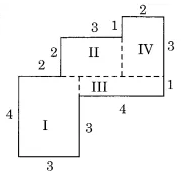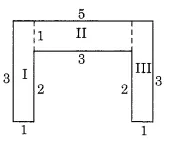# Q 10.     By splitting the following figures into rectangles, find their areas (The measures are given in centimetres).(a) Split the given figures into small rectangular parts as shown here;Now, the area of the rectangle (I) =  = 4  3 = 12
the area of the rectangle (II) =  = 3  2= 6
the area of the rectangle (III) =  = 4  1 = 4
the area of the rectangle (IV) =  = 3  2 = 6

So, the total area of the figure = sum area of all rectangles
= (12 + 6 + 4 + 6)
= 28

(b) Split the given figure into smalle rectangles  as shown here;the area of the rectangle (I) =  = 3  1 = 3
the area of the rectangle (II) =  = 3  1= 3
the area of the rectangle (III) =  = 3  1 = 3 ?

Therefore, the total area of the figure = sum of the area of all the small rectangles
=
=

## Related Chapters

### Preparation Products

##### JEE Main Rank Booster 2021

This course will help student to be better prepared and study in the right direction for JEE Main..

₹ 13999/- ₹ 9999/-
##### Rank Booster NEET 2021

This course will help student to be better prepared and study in the right direction for NEET..

₹ 13999/- ₹ 9999/-
##### Knockout JEE Main April 2021 (Easy Installments)

An exhaustive E-learning program for the complete preparation of JEE Main..

₹ 4999/-
##### Knockout NEET May 2021

An exhaustive E-learning program for the complete preparation of NEET..

₹ 22999/- ₹ 14999/-